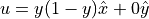# Configuration File Syntax

The following syntax should be followed when creating configuration files or OpenCMP will have trouble parsing them.

## Basic Parameter Specification

The most basic configuration file syntax has a header, indicated by square brackets, followed by various parameters whose values are assigned using “=”.

[HEADER]
param1 = value1
param2 = value2


For the standard header’s and parameter options see Configuration File Options Guide.

Parameters can have a single value - like the example above - or can have multiple values given as a comma-separated list.

[HEADER]
param3 = value3a, value3b, value3c


## Multi-Level Parameters

Some parameters have multiple levels, which requires the use of a new assignment symbol “->”.

As an example, consider a Stokes flow problem that will use an HDiv finite element space for velocity and an L2 finite element space for pressure. This would be specified as follows:

[FINITE ELEMENT SPACE]
elements = u -> HDiv
p -> L2


## Whitespace

In general, whitespace is ignored when parsing the configuration files. The major exceptions are filepaths and multi-level parameters.

Filepaths that include whitespace must contain that whitespace when specified in the configuration files.

Warning

There is a known issue with the configuration file parser that will cause it to remove all whitespace when reading certain parameter values. It is preferable avoid whitespace in filepaths.

Multi-level parameters must use a newline to separate different values and must have some form of indentation on subsequent lines.

[WORKS]
param = var1 -> value1
var2 -> value2

[ALSO WORKS]
param = var1 -> value1
var2 -> value2

[NEEDS A NEWLINE]
param = var1 -> value1 var2 -> value2

[NEEDS AN INDENT]
param = var1 -> value1
var2 -> value2


## Mathematical Expressions

OpenCMP supports the use of standard mathematical notation and follows standard order of operations.

The following operators can be used:

• “-” - subtraction

• “*” - multiplication

• “/” - division

• “^” - exponentiation

• “()” - denotes order of operations

The following constants are defined:

• “pi” -• “e” - Euler’s number

The following functions are defined:

• “sin()” - sine function in radians

• “cos()” - cosine function in radians

• “tan()” - tangent function in radians

• “sig()” - sigmoid function

• “H()” - Heaviside function

• “exp()” - exponential function

• “abs()” - absolute value function

• “trunc()” - truncates the input

• “round()” - rounds the input

• “sqrt()” - square root function

• “sgn()” - returns the sign of the input

• “ramp()” - cosine ramp

The following variables are defined by default for the given simulation domain. Model variables are also defined according to the model chosen. For example, a Stokes flow problem would have “u” and “p” defined as model variables.

• “x”, “y”, “z” - spatial coordinates

• “t” - temporal coordinate

Additional known variables can be added through the “parameter_names” option in the main configuration file. For example, if the diffusion coefficient is dependent on the viscosity then the main configuration file should include the following:

[OTHER]
parameter_names = kinematic_viscosity_all


Then, the model configuration file could contain an expression for the diffusion coefficient in terms of viscosity:

[PARAMETERS]
diffusion_coefficients = a -> 2.0 * kinematic_viscosity_all ^ 2


### Coordinates

Coordinates are indicated by “<>”. For example, specifying the origin as the centroid for a diffuse interface mask would be done as follows:

[CENTROIDS]


Note

Coordinates should only ever have numerical values. They can’t contain mathematical expressions.

### Vectors

Vector expressions are indicated by “[]”. Unlike coordinates, vector expressions can contain mathematical expressions. However, the length of the vector expression must match the model and mesh dimensions or issues will arise.

As an example, a Stokes flow velocity boundary condition for a 2D domain could be specified as follows:

[DIRICHLET]
u = left -> [y*(1 - y), 0.0]


which would evaluate as.

## Importing Python Functions

Parameter values can be obtained from imported Python functions in cases where their mathematical expressions require operators not available to the OpenCMP parser or require the use of external Python libraries.

Consider the following example:

[DIRICHLET]
u = left -> IMPORT(left_bc_func)


IMPORT indicates that the boundary condition value will be obtained by importing a Python function “left_bc_func”. This function is defined in the Python script “import_functions.py” which should be placed in the main simulation directory. The contents of “import_functions.py” would appear as follows:

def left_bc_func(t_param, model_variables, mesh):
# Some code
return u_left_value


The function to call must have the name given within IMPORT, must take the four indicated arguments, and must return a grid function. The arguments passed to the function are as follows:

• t_param - A list of time parameters for each time step in the time discretization scheme in reverse order. For example, if the implicit Euler time discretization scheme is being used t_param = [t^n+1, t^n] where t^n+1 is the time parameter for the time step being solved for and t^n is the time parameter for the last solved time step.

• model_variables - A list of dictionaries where each dictionary contains the value of each model variable. These values are given for the time step associated with the order in the list. For example, if the implicit Euler time discretization scheme is being used with the Poisson equation model_variables = [{‘u’: u^n+1}, {‘u’: u^n}]. Note that model_variables will contain any parameters with variable value given by the “parameter_names” parameter in the main configuration file (see Configuration File Options Guide).

• mesh - The mesh used by the simulation.

• time_step - An integer indicating which time step the returned value should be calculated for. For example, time_step = 0 means the returned value should be calculated for time t_param.